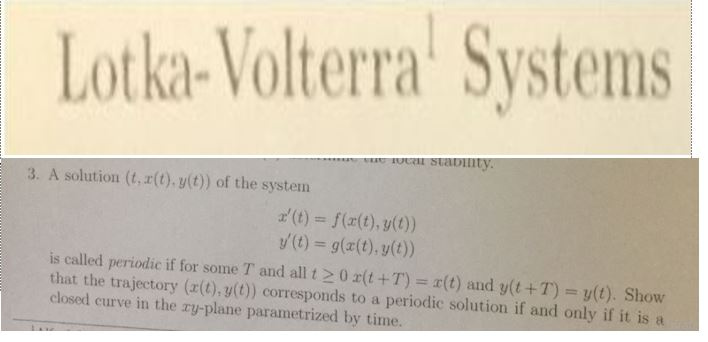# Lotka-Volterra Systems3. A solution (t,r(t). y(t)) of the system(t)f((t),yt)y(t) = g(x(t), y(t))is called periodic if for some T and all t > 0 x(t +T) = x(t) and y(t + T) = y(t). Showthat the trajectory (a(t),g() corresponds to a periodic solution if and only if it isclosed curve in the ry-plane parametrized by time.

Question
92 views

pls explain to me step by step. thankshelp_outlineImage TranscriptioncloseLotka-Volterra Systems 3. A solution (t,r(t). y(t)) of the system (t)f((t),yt) y(t) = g(x(t), y(t)) is called periodic if for some T and all t > 0 x(t +T) = x(t) and y(t + T) = y(t). Show that the trajectory (a(t),g() corresponds to a periodic solution if and only if it is closed curve in the ry-plane parametrized by time. fullscreen
check_circle

Step 1

To establish the (given) necessary and sufficient conditions for the periodicity of solutions of the given system

Step 2

Point to note in the problem: the fact that x(t) and y(t) are solutions of a system (of differential equations) is not relevant to the periodicity to be discussed.

Step 3

### Want to see the full answer?

See Solution

#### Want to see this answer and more?

Solutions are written by subject experts who are available 24/7. Questions are typically answered within 1 hour.*

See Solution
*Response times may vary by subject and question.
Tagged in

### Math